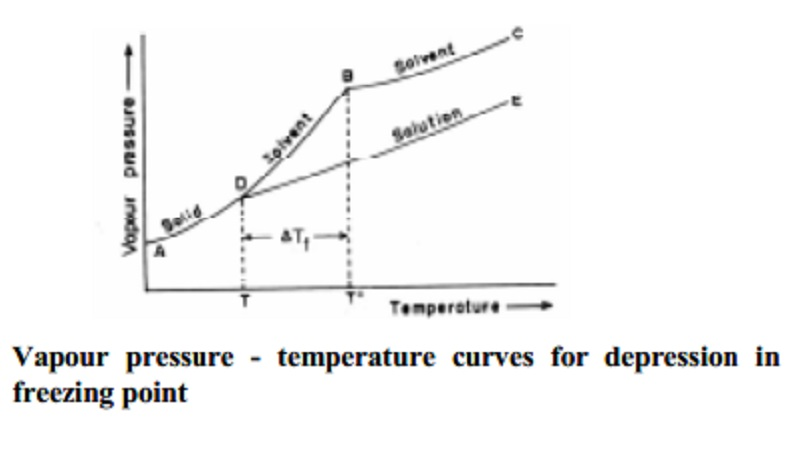Home | | Chemistry | | Chemistry | Depression of freezing point of dilute solution

# Depression of freezing point of dilute solutionDepression of freezing point of dilute solution , Determination of molecular weight from depression in freezing point, cryoscopic constant (or) molal freezing point depression constant..Freezing point is the temperature at which solid and liquid states of a substance have the same vapour pressure.

Depression of freezing point of dilute solution

Freezing point is the temperature at which solid and liquid states of a substance have the same vapour pressure. According to Raoult's law, addition of a non-volatile solute to solvent lowers the vapour pressure of the solvent and hence, the vapour pressure of pure solvent is greater than the vapour pressure of solution. Thus the temperature at which the solution and its solid form existing in equilibrium and possessing the equal vapour pressures, is lowered. That is, the freezing point of solution is lowered. The lowering of the freezing point of the solution from that of the freezing point of the pure solvent is known as depression in freezing point of the solution.

Consider the vapour pressure curves shown in Fig.. Generally when the temperature of a solid substance that is used as the solvent is raised, the vapour pressure also raises. AB curve depicts this. Similarly curve BC represents the increase in vapour pressure of the liquid solvent with increase in temperature. Curves AB and BC meet at B corresponding to To temperature which is the freezing point of the pure solvent. At To, the vapour pressure of the liquid and solid states of the solvent are equal at B. Since the vapour pressure of the solution is always lower than that of its pure solvent, the vapour pressure curve of the solution DE always lie below that of the pure solvent.

D is the point of intersection of the vapour pressure curves of solution and pure solvent. The temperature at D is the freezing point of the solution and is seen to be lower than To. The depression in freezing point is ∆Tf=T0-T. The measured depression in freezing point (∆Tf) is found to be directly proportional to the molality (m) of the solute in solution. That is, ∆Tf  props to m or ∆Tf - Kfm , where Kf is called s the the cryoscopic constant (or) molal freezing point depression constant. `Kf' is defined as the depression in freezing point produced when one mole of solute is dissolved in 1 kg solvent. It is also the depression in freezing point of one molal solution. Freezing point depression of a dilute solution is found to be directly proportional to the number of moles (and hence the no.of molecules) of the solute dissolved in a given amount of the solvent. Also ∆Tf is independent of the nature of the solute as long as it is non-volatile. Hence depression in freezing point is considered as a colligative property.

Determination of molecular weight from depression in freezing point

∆Tf = Kf.m

Where m = molality

M = n2 / W1   nad  n2 = W2  / M2

W1 =      Weight of the solvent in Kg;

M2 =      Molecular weight of solute

m = W2 / M2W1

∆Tf  = Kf. (W2 / M2W1)

M2 = Kf W2 / ∆Tf W1  -   K.kg mol-1g/ K.kg

M2 = Kf W2 / ∆Tf W1  -   g mol-1

Thus the molecular weight of the solute can be calculated.Study Material, Lecturing Notes, Assignment, Reference, Wiki description explanation, brief detail

Related Topics